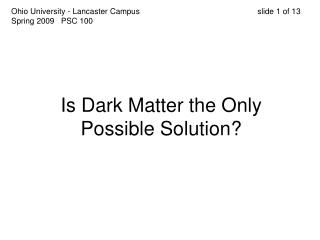DownloadDownload PresentationIs Dark Matter the Only Possible Solution?

# Is Dark Matter the Only Possible Solution?

Download Presentation## Is Dark Matter the Only Possible Solution?

- - - - - - - - - - - - - - - - - - - - - - - - - - - E N D - - - - - - - - - - - - - - - - - - - - - - - - - - -
##### Presentation Transcript

1. Ohio University - Lancaster Campus slide 1 of 13Spring 2009 PSC 100 Is Dark Matter the OnlyPossible Solution?

2. Ohio University - Lancaster Campus slide 2 of 13Spring 2009 PSC 100 In 1983, Mordehai Milgrom, a professor at the Weismann Institute in Israel, proposed a startling alternative to dark matter. It was a revision of Newton’s 2nd Law of Motion. Milgrom called it MOND: Modified Newtonian Dynamics www.weizmann.ac.il

3. Ohio University - Lancaster Campus slide 3 of 13Spring 2009 PSC 100 Newton’s Law of Gravity states that the force between two objects comes from the product of their masses, and is proportional to the inverse of the square of the distance between them… Force = Gm1m2 r2 G is a proportionality constant, 6.67 x 10-11m3/kg▪s2 The fact that G is so small indicates that gravity is a weak force.

4. Ohio University - Lancaster Campus slide 4 of 13Spring 2009 PSC 100 Newton’s 2nd Law also states that a force on an object is given by the object’s mass and the acceleration applied to it… F = m▪a

5. Ohio University - Lancaster Campus slide 5 of 13Spring 2009 PSC 100 What Milgrom proposed was that when the distance between two objects was very large, then the acceleration would be very small, but not as small as Newton predicted. Newton’s 2nd law changes to… F = m▪a2 a0 where a0 is 1.2 x 10-10 m/s2

6. Ohio University - Lancaster Campus slide 6 of 13Spring 2009 PSC 100 a0 is a constant derived from the speed of light and the age of the universe. If an object had started at rest and accelerated with an acceleration a0 since the beginning of the universe, today it would be moving at c, the speed of light, 3.00 x 108 m/s. More importantly… then F = Gm1m2= ma2 r2 a0

7. Ohio University - Lancaster Campus slide 7 of 13Spring 2009 PSC 100 Since, over great distances, the gravitational force is proportional to a2, not just a, less mass is needed to account for the force. In other words, Milgrom’s equation eliminates the need for dark matter.

8. Ohio University - Lancaster Campus slide 8 of 13Spring 2009 PSC 100 How does Milgrom’s equation eliminate the need for dark matter in our galaxy? Diagram Credit: Phil Hibbs, Michael Gmirkin

9. Ohio University - Lancaster Campus slide 9 of 13Spring 2009 PSC 100 Gm1m2= ma2 r2 a0 Let’s substitute in mgalaxy for m1 and msun for m2 and the m on the right, then we can cancel out the msun on both sides: GM = a2 r2 a0

10. Ohio University - Lancaster Campus slide 10 of 13Spring 2009 PSC 100 GMa0 = a2 Now take the square r2 root of both sides: GMa0= a r

11. Ohio University - Lancaster Campus slide 11 of 13Spring 2009 PSC 100 Finally, set this equation equal to the equation that relates velocity to acceleration for a star in a circular orbit around the galaxy’s center: a = GMa0 = v2 r r and cancel out the r’s on both sides: GMa0 = v2

12. Ohio University - Lancaster Campus slide 12 of 13Spring 2009 PSC 100 Take the square root of both sides: GMa0 = v What we understand from this, is that the velocity of a star orbiting the center of the galaxy has no dependence on its distance, r, from the center of the galaxy. And NO dark matter was needed. 4

13. Ohio University - Lancaster Campus slide 13 of 13Spring 2009 PSC 100 Does this mean that the idea of dark matter is worthless? Many astrophysicists agree that Milgrom’s equation predicts and describes the rotational behavior of the galaxy (and many other low acceleration situations also!) But most astrophysicists don’t agree that the equation explains the cause of the behavior. Most astrophysicists remain convinced that the equation just coincidentally coincides with the behavior of dark matter.What do you think?